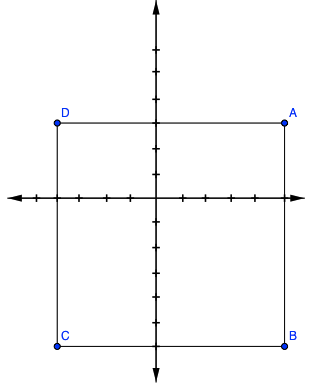### Home > GC > Chapter Ch1 > Lesson 1.2.3 > Problem1-73

1-73.

Plot the following points on another sheet of graph paper and connect them in the order given. Then connect points $A$ and $D$. Homework Help ✎

$A(5, 3), B(5, −6), C(−4, −6)$, and $D(−4, 3)$

1. What is the resulting figure?

2. Find the area of shape $ABCD$.

3. If $ABCD$ is rotated $90°$clockwise ($\circlearrowright$) about the origin to form $A^\prime B^\prime C^\prime D^\prime$, what are the coordinates of the vertices of $A^\prime B^\prime C^\prime D^\prime$?$A' (3, −5), B' (−6, −5), C' −6, 4), D' (3,4)$